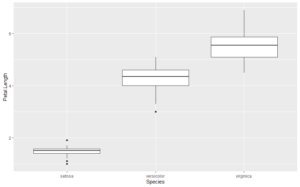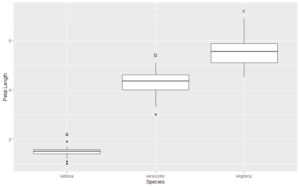## Compact Letter Displays

Compact letter displays (CLDs) are letters that show which treatment groups are not significantly different by some statistical test. It is often desirable to include CLDs on graphs. Here I show how to add them to a box plot created with ggplot2.

First, make an example plot using the iris data:

```library(ggplot2)
library(car)
library(QsRutils)
data("iris")
plt1 <- ggplot(data = iris, aes(x=Species, y=Petal.Length)) + geom_boxplot()
plt1```I want to add CLDs just above the tops of the box whiskers in the plot. I can use the base boxplot function to capture the coordinates for these points.

```box.rslt <- with(iris, graphics::boxplot(Petal.Length ~ Species, plot = FALSE))
str(box.rslt)
List of 6
\$ stats: num [1:5, 1:3] 1.1 1.4 1.5 1.6 1.9 3.3 4 4.35 4.6 5.1 ...
\$ n    : num [1:3] 50 50 50
\$ conf : num [1:2, 1:3] 1.46 1.54 4.22 4.48 5.37 ...
\$ out  : num [1:2] 1 3
\$ group: num [1:2] 1 2
\$ names: chr [1:3] "setosa" "versicolor" "virginica"```

The fifth row of `box.rslt\$stats` gives the y coordinates for the tops of the whiskers.

```box.rslt\$stats
[,1] [,2] [,3]
[1,] 1.1 3.30 4.50
[2,] 1.4 4.00 5.10
[3,] 1.5 4.35 5.55
[4,] 1.6 4.60 5.90
[5,] 1.9 5.10 6.90```

Next I have to get a vector of the letters to add to the plot. For this example, I will make a pairwise t-test which outputs a matrix of p-values.

`ptt.rslt <- with(iris, pairwise.t.test(Petal.Length, Species, pool.sd = FALSE))`

Looking at the structure of `ptt.rslt`, we see that `ptt.rslt\$p.value` gives a matrix of p.values:

```str(ptt.rslt)

List of 4
\$ method : chr "t tests with non-pooled SD"
\$ data.name : chr "Petal.Length and Species"
\$ p.value : num [1:2, 1:2] 1.99e-45 2.78e-49 NA 4.90e-22
..- attr(*, "dimnames")=List of 2
.. ..\$ : chr [1:2] "versicolor" "virginica"
.. ..\$ : chr [1:2] "setosa" "versicolor"
- attr(*, "class")= chr "pairwise.htest"

ptt.rslt\$p.value

setosa      versicolor
versicolor 1.986887e-45 NA
virginica 2.780888e-49 4.900288e-22```

From this matrix we can use `QsRutils::make_letter_assignments` to get a vector of letters for our CLDs.

```ltrs <- make_letter_assignments(ptt.rslt)
str(ltrs)

List of 3
\$ Letters          : Named chr [1:3] "a" "b" "c"
..- attr(*, "names")= chr [1:3] "setosa" "versicolor" "virginica"
\$ monospacedLetters: Named chr [1:3] "a  " " b " "  c"
..- attr(*, "names")= chr [1:3] "setosa" "versicolor" "virginica"
\$ LetterMatrix     : logi [1:3, 1:3] TRUE FALSE FALSE FALSE TRUE FALSE ...
..- attr(*, "dimnames")=List of 2
.. ..\$ : chr [1:3] "setosa" "versicolor" "virginica"
.. ..\$ : chr [1:3] "a" "b" "c"
- attr(*, "class")= chr "multcompLetters"

ltrs\$Letters
setosa versicolor  virginica
"a"        "b"        "c"```

`ltrs\$Letters` gives the vector of letters that we want. Now we can make a data frame to add the CLDs to the box plot.

```x <- c(1:length(ltrs\$Letters))
y <- box.rslt\$stats[5, ]
cbd <- ltrs\$Letters
ltr_df <- data.frame(x, y, cbd)
ltr_df
x   y cbd
setosa     1 1.9   a
versicolor 2 5.1   b
virginica  3 6.9   c

```

If we plot the CLDs at the coordinates in `ltr_df`, they will over plot the tops of the whiskers . We need to nudge the CLDs upward to avoid the overlap. To determine how much to nudge, I will get the range of the Y-axis and nudge upward 5% of this range.

```lmts <- get_plot_limits(plt1)
y.range <- lmts\$ymax - lmts\$ymin
y.nudge <- 0.05 * y.range
plt1 +
geom_text(data = ltr_df, aes(x=x, y=y, label=cbd), nudge_y = y.nudge)

```The CLDs are perfectly positioned, and without any trial and error.

## Get ggplot plot panel limits

I have added a new function (`get_plot_limits`) to my package `QsRutils`.  It extracts the minimum and maximum X and Y values for a ggplot panel. This is useful in formatting ggplots. For example, you may wish to expand the panel to avoid text running out of the panel, or nudge text relative to some point. For an example, see my post on adding compact letter displays to box plots created with `ggplot2`.

## Rotatable 3D Plots

The R package vegan includes the function ordiplot for making ordination plots using R’s base graphics. Additionally vegan provides several functions for enhancing the plots with spiders, hulls, and ellipses. It is even possible to overlay an ordination plot with a cluster diagram. (See my package ggordiplots on GitHub for making the same kinds of plots with ggplot graphics.) Vegan’s functions for adding these layers begin with “ordi”: ordispider, ordihull, ordiellipse, ordicluster. Earlier this year I discovered the package vegan3d. It makes use of rgl graphics which means that the plots it generates can be scaled and rotated with the mouse. This is not just fun – it allows you to see how well separated treatment groups are in the ordination space.

Vegan3d’s function ordirgl is similar to ordiplot – it draws the ordination plot depicting samples and/or species. Other functions beginning with “orgl” are similar to the ones beginning with “ordi” in vegan: orglpoints, orglspider, orglellipse, orglcluster. See the vegan3d documentation for full instructions on how to use these.

I made an interactive html page of a slightly enhanced version of the example given in the vegan3d documentation, viewable if you CLICK HERE. Some explanation of the last code block of the Rmarkdown file reproduced here is necessary.

```open3d() # Opens a graphic device for the plot.
## wgl
##   8
par3d(windowRect = c(20, 40, 1000, 1200)) # left, top, right, bottom in pixels
my.color <- c("red", "blue")
ordirgl(ord, size=4, col = my.color[as.numeric(sam\$Type)]) # plot points
with(sam, orglspider(ord, Type, col = my.color[1:2], scaling = "sites")) # add spiders
with(sam, orglellipse(ord, Type, col = my.color[1:2], kind = "se", conf = 0.95, scaling = "sites")) # add ellipses
rglwidget() # You cannot run this line in RStudio.```

The open3d command opens an rgl graphics window. If you are using RStudio, this window will open outside of RStudio. The next 5 lines build the plot in the graphics window. At any step after the line beginning with ordirgl you can zoom and rotate the plot in the graphics window. The last line, rglwidget(), is necessary to knit the html file. If you try to run it in RStudio, it will cause RStudio to freeze. If you try to use the RStudio menu to knit the html document, RStudio will freeze. So DO NOT do either of these things!

To knit the Rmarkdown file into an html file, you must enter the command in the console window. In this case the command is:

`rmarkdown::render(input = "vegan3d_example.Rmd", output_format = "html_document", output_file = "vegan3d_example.html")`